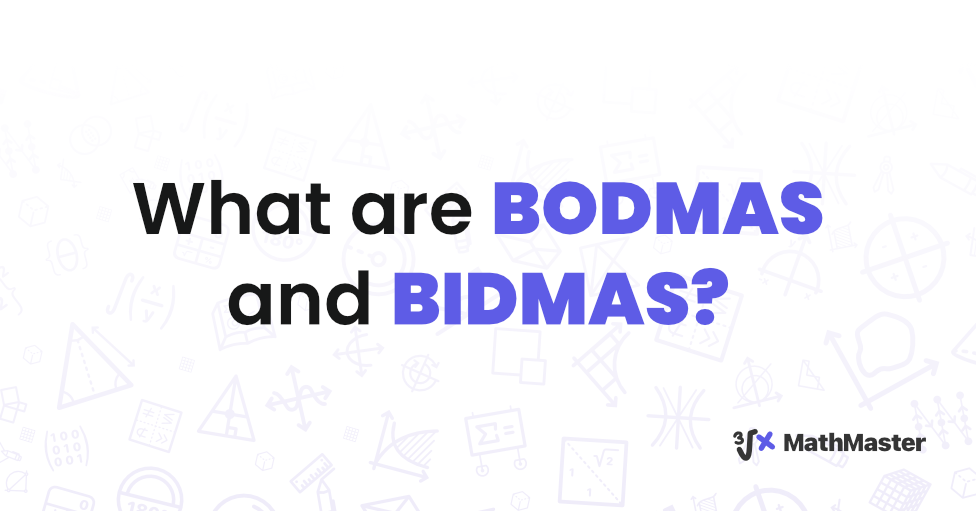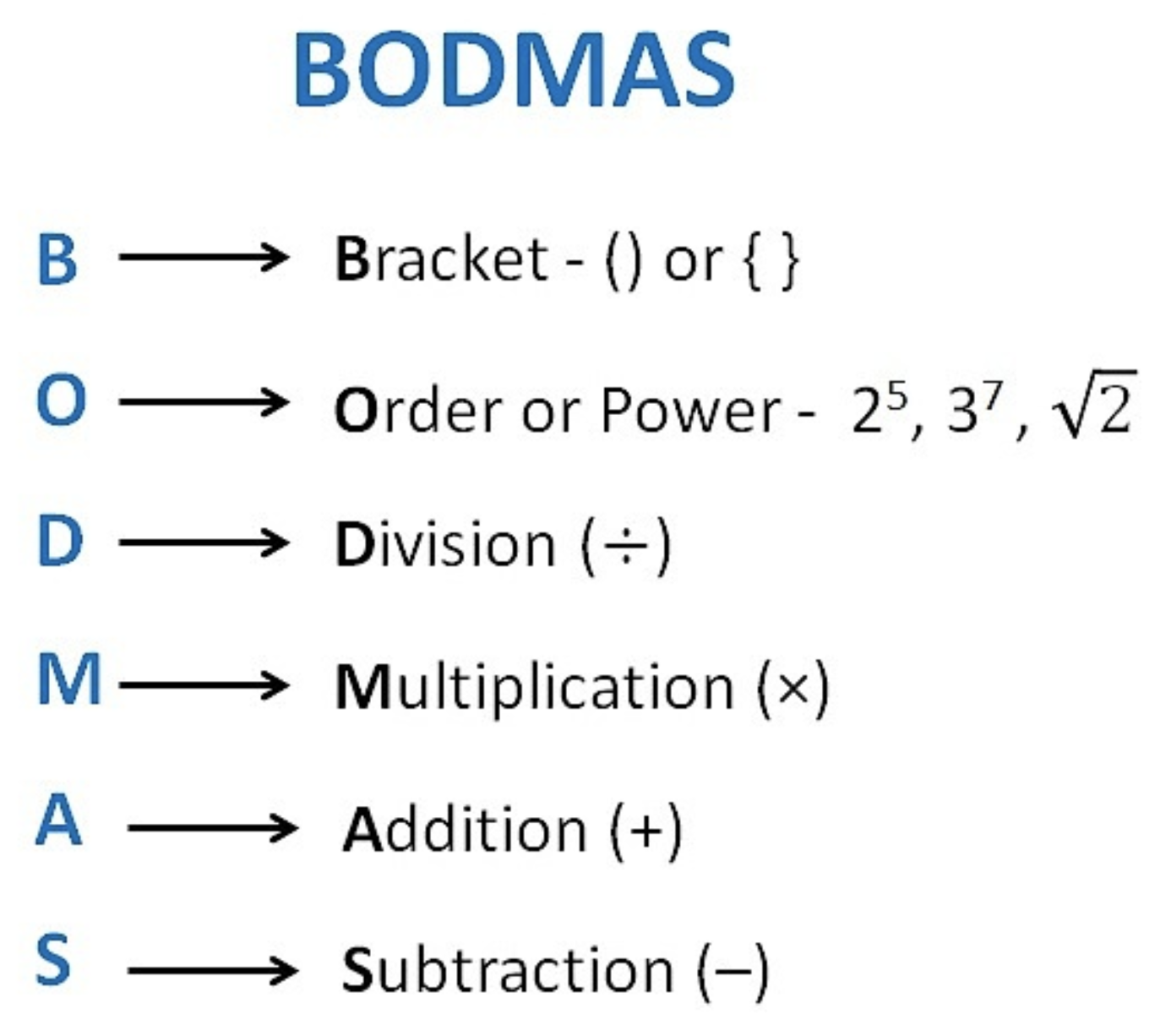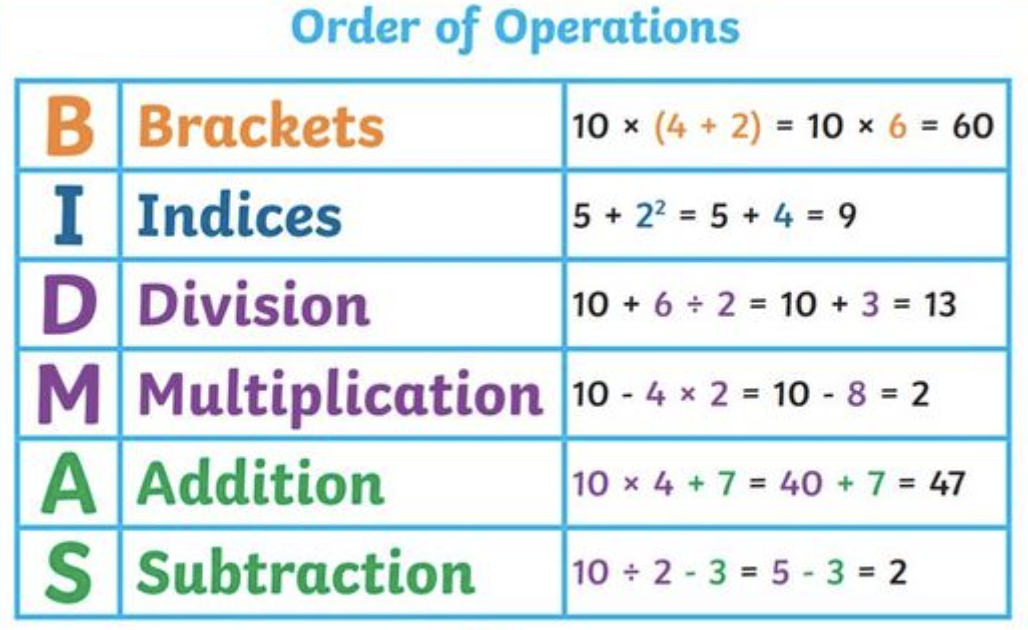# What Is BODMAS and BIDMAS?In the realm of mathematics, the proper order of operations is like the foundation of a building – it provides the necessary structure for complex calculations to be carried out accurately. Two acronyms that often come to the forefront when discussing these mathematical structures are the BODMAS rule and the BIDMAS rule. These acronyms serve as guidelines for determining the sequence in which different mathematical operations are performed within a given expression.

## Understanding BODMAS RuleBODMAS is a mnemonic that stands for Brackets, Orders, Division/Multiplication, and Addition/Subtraction. It is a widely recognized rule that dictates the sequence in which mathematical operations should be performed to achieve accurate results.

1. Brackets: The first step involves solving expressions enclosed within brackets. This can include parentheses , square brackets [], or curly braces {}. The operations within the innermost set of brackets should be performed first.
2. Orders: Orders refer to operations involving powers and roots. Any exponentiation or square root calculations should be done following the bracket evaluations.
3. Division/Multiplication: After tackling the brackets and orders, the next step is to perform any division or multiplication operations from left to right in the given expression.
4. Addition/Subtraction: The final step involves addition and subtraction, again carried out from left to right. These operations should be performed after addressing brackets, orders, and division/multiplication.

## BODMAS Example

The BODMAS rule ensures that expressions are solved systematically, preventing ambiguity and generating consistent results. Let's consider an example to illustrate the application of what is BODMAS in maths:

Example: Solve the expression 5 + 8 * $12 ÷ 4$ - 32.
1. Brackets: Inside the parentheses, 12 ÷ 4 equals 3. So, the expression becomes 5 + 8 * 3 - 32.
2. Orders: The square of 3 is 9. The expression now reads 5 + 8 * 3 - 9.
3. Division/Multiplication: Multiplying 8 by 3 gives 24. The expression becomes 5 + 24 - 9.
4. Addition/Subtraction: Finally, 5 + 24 equals 29, and subtracting 9 yields 20.

Following the BODMAS sequence ensures that the expression is evaluated correctly, and the result is 20.

## Understanding BIDMAS RuleWhile the BODMAS rule is widely used in many regions, particularly in the United States and the United Kingdom, BIDMAS is the counterpart acronym mainly employed in the United Kingdom. BIDMAS stands for Brackets, Indices, Division/Multiplication, and Addition/Subtraction. The key distinction here is the use of the term "Indices" instead of "Orders."

1. Brackets: As in BODMAS questions, the first step is to address any operations within brackets, beginning with the innermost set.
2. Indices $Orders$: The term "Indices" refers to operations involving powers and roots, similar to the "Orders" component in BODMAS. This step involves evaluating any exponentiation or square root calculations.
3. Division/Multiplication: Like in BODMAS, this step requires division and multiplication operations from left to right.
4. Addition/Subtraction: The final step involves addition and subtraction, again performed from left to right.

To better comprehend the BIDMAS process, let's revisit the example we used earlier:

Example: Solve the expression 5 + 8 * $12 ÷ 4$ - 32.

1. Brackets: As before, 12 ÷ 4 equals 3, resulting in the expression 5 + 8 * 3 - 32
2. Indices: The square of 3 remains 9. The expression reads 5 + 8 * 3 - 9.
3. Division/Multiplication: Multiplying 8 by 3 still gives 24. The expression becomes 5 + 24 - 9.
4. Addition/Subtraction: Finally, 5 + 24 equals 29, and subtracting 9 yields 20, just as in the BODMAS rule approach.

## BODMAS and BIDMAS: A Brief Historical Overview

BODMAS and BIDMAS origins date way back to the early development of mathematical notation. These rules evolved over centuries as a response to the need for standardized procedures in mathematical calculations. While the exact inception dates remain unclear, BODMAS gained prominence in various countries, including the United States. At the same time, BIDMAS emerged as its counterpart in the United Kingdom. Both acronyms are rooted in the need for a consistent order of operations to prevent mathematical ambiguity and ensure accurate results. Over time, these rules have become fundamental pillars of arithmetic and algebraic problem-solving, serving as indispensable tools in mathematics education.

## MathMaster Your BODMAS and BIDMAS Mathematical Companion

If you grapple with understanding BODMAS and BIDMAS questions, here's the solution. A powerful math-solving tool called MathMaster is here to assist you. MathMaster is designed to help you navigate complex mathematical expressions and ensure accurate results. With its user-friendly interface and step-by-step guidance, MathMaster can simplify your mathematical journey and clarify concepts like 'What is BODMAS in maths?' Whether you're a student, a professional, or an enthusiast, MathMaster is your reliable companion for confidently conquering mathematical challenges.

## Conclusion

BODMAS rule and BIDMAS rule are crucial structures that guide the proper execution of mathematical calculations. Both acronyms emphasize the importance of adhering to a systematic sequence of operations – whether involving brackets, indices, division, multiplication, addition, or subtraction – to achieve accurate and reliable results. So, whether you're BODMAS questions or BIDMAS questions, the journey might have slightly different names, but the destination remains the same: precise mathematical solutions.

### FAQ

Do BODMAS and BIDMAS always result in the same answer? Yes, when applied correctly, both BODMAS and BIDMAS should always yield the same answer for a given mathematical expression. While these acronyms use slightly different terminology $Orders in BODMAS and Indices in BIDMAS$, the sequence of operations they dictate remains consistent.
Can BODMAS and BIDMAS be applied to complex equations? Absolutely. BODMAS and BIDMAS are fundamental rules that can be applied to complex equations involving multiple operations and parentheses. By following the prescribed sequence of brackets, indices/orders, division/multiplication, and addition/subtraction, these rules help maintain clarity and accuracy in solving even the most intricate mathematical expressions.
What is the purpose of BODMAS and BIDMAS? BODMAS and BIDMAS are acronyms that outline the correct sequence for performing mathematical operations within an expression. They ensure consistency in calculations by providing a systematic order of operations, preventing ambiguity, and generating accurate results.